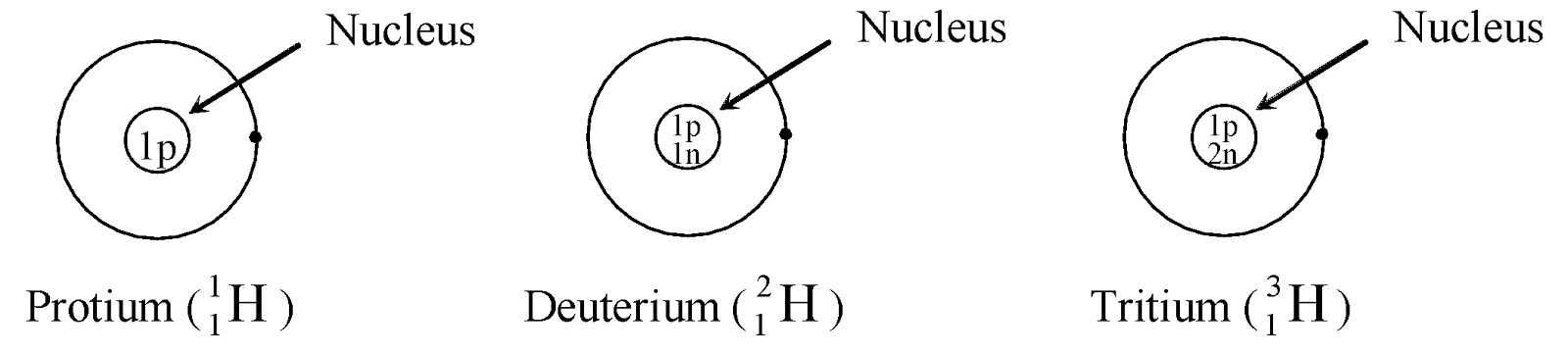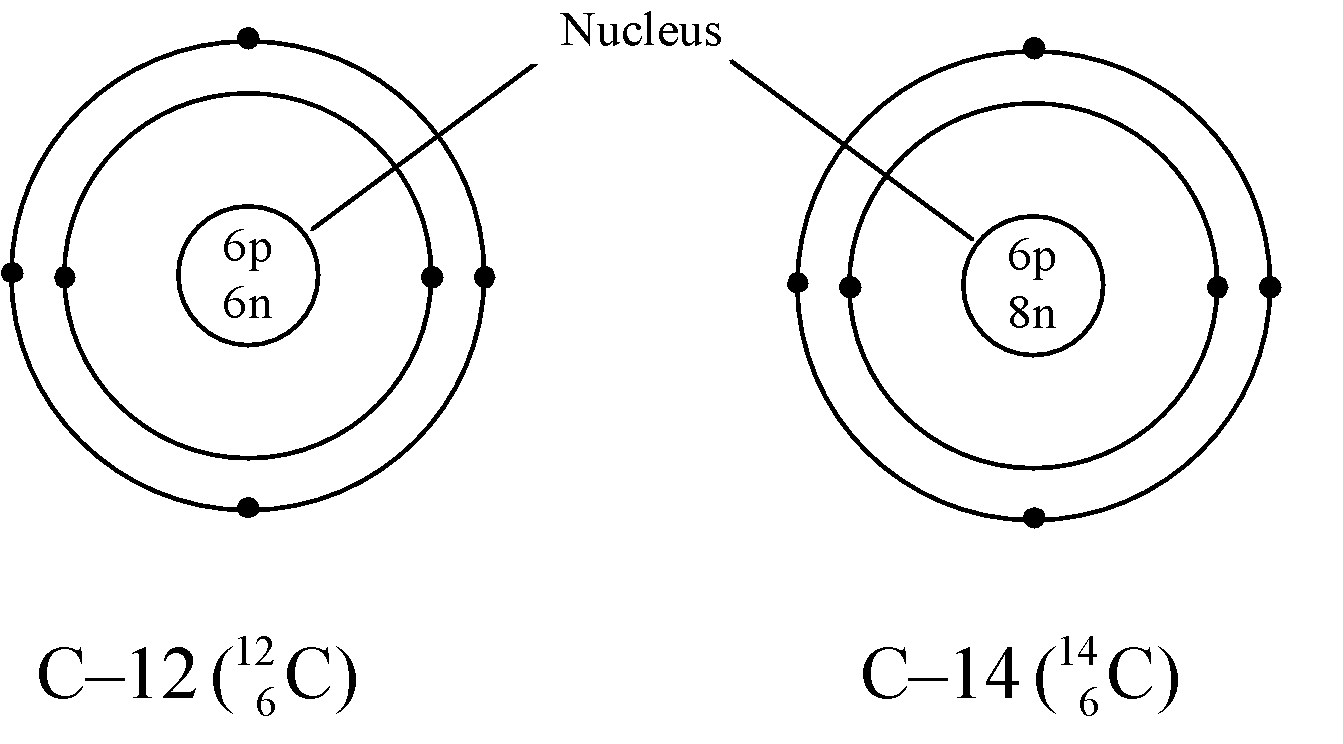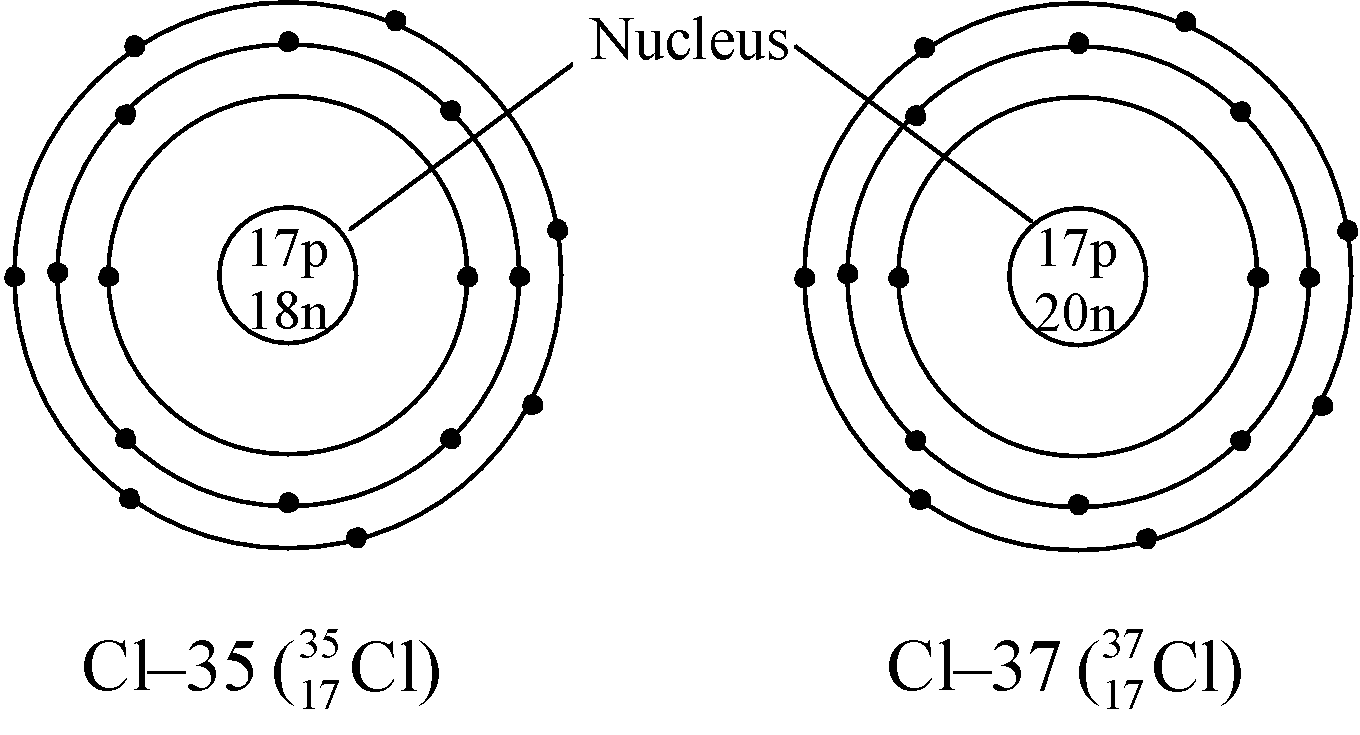# ISOTOPES

## Structure of atom of Class 9

In nature, there are several elements, whose atoms have the same atomic number but different mass number, such atoms are known as isotopes and can be defined as:

“Isotopes are the atoms of the same element, having the same atomic number but different mass number”.

As, it is known that

Atomic number (Z) = Number of protons = Number of electrons

So we may conclude that isotopes contain the same number of electrons as well as protons, and we know that

Mass number = Number of protons + Number of neutrons

But as we know that number of protons in them are equal so we can conclude that isotopes of an element differ only in the number of neutrons present in the nucleus. This can be understood more clearly by the following examples:

Ex. Isotopes of Hydrogen: There are three isotopes of hydrogen, namely protium, deuterium and tritium.

They have only one proton, but differ in the number of neutrons and contain 0, 1, 2 neutrons in their nucleus respectively.Isotope Atomic No. Mass No. No. of protons No. of neutrons No. of electrons1 1 1 1 – 1 = 0 11 2 1 2 – 1 = 1 11 3 1 3 – 1 = 2 1

Ex. Isotopes of Carbon: Carbon has mainly two isotopes which are as follows:

 Isotope Atomic No. Mass No. No. of protons No. of neutrons No. of electrons6 12 6 12 – 6 = 6 66 14 6 14 – 6 = 8 6Ex. Isotopes of Chlorine: There are two isotopes of chlorine which are as follows:

 Isotope Atomic No. Mass No. No. of protons No. of neutrons No. of electrons17 35 17 35 – 17 = 18 1717 37 17 37 – 17 = 20 17### GENERAL CHARACTERISTICS OF ISOTOPES:

• Same atomic number:  The isotopes of an element have the same atomic number i.e. they have same number of protons and same number of electrons.
• Different mass number: They have different mass number and hence differ in the number of neutrons present in the nucleus.
• Same chemical properties: They have same chemical properties as they have same number of electrons and therefore same electronic configuration and valence electrons.
• Different physical properties: Since they have different mass number hence they differ in their physical properties such as melting point, boiling point, density, etc.
• Different nuclear properties: Due to the difference in the number of neutrons in their nucleus they show different nuclear properties e.g. C–14 isotope of carbon is radioactive whereas C–12 isotope is non-radioactive. The radioactive isotope of an element is known as radioisotope.

### FRACTIONAL ATOMIC MASSES AND CALCULATION OF AVERAGE ATOMIC MASSES:

The mass of an atom of any natural element is taken as the average mass of all the naturally occurring atoms of that element. Hence, if an element has no isotope then the atomic mass of its atom would be the same as its mass number. But if an element occurs in isotopic form, then we have to know the percentage of each isotopic form to calculate its average atomic mass.

Ex. Calculation of average atomic mass can be explained with the help of the following example:

In nature, the two isotopic forms of chlorine viz.,andare found in the ratio of 3:1. Hence,

Average atomic mass ==== 35.5 u

This does not mean that any one atom of chlorine has a fractional mass of 35.5 u. It means that if we take a certain amount of chlorine, which consists of both the isotopes of chlorine, then it will have the average atomic mass of 35.5 u. The reason for those fractional atomic masses is that for an element existing in different isotopes, i.e., atoms with different mass numbers, the atomic mass of the elements is the average value which comes out to be fractional.

### APPLICATIONS OF ISOTOPES:

• In agriculture: Certain elements such as boron, cobalt, copper, manganese, zinc and molybdenum are necessary is very minute quantities for plant nutrition.By radioactive isotopes we can identify the presence and requirements of these element in the nutrition of plants.
• In industry: Coating on the arm of clock to seen in dark. To identify the cracks in metal casting.
• In medicine : Thyroid, bone diseases, brain tumours and cancer and diagnosed, controlled or destroyed with the help of radioactive isotopes like,Na, iodine, phosphorus etc.
•  Determination of the mechanism of chemical reaction: By replacing an atom or molecule by its isotope.
•  In carbon dating : Will and Libby (1960) developed the technique of radiocarbon dating to determine the age of plants, fossils and archeological samples.

Isotopes (Like Uranium - 238) are used in nuclear reactor to produce energy and power.